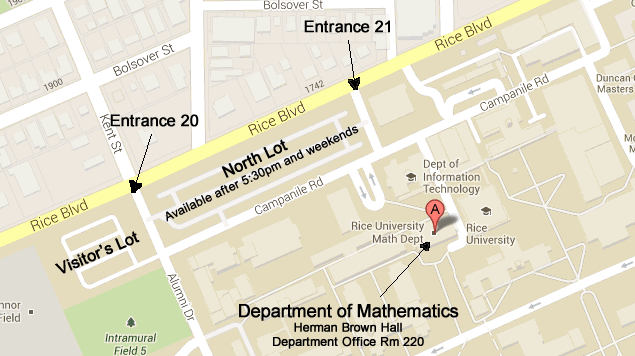## Partial differential equations

### Faculty

• Robert Hardt (Emeritus)

Geometric measure theory, partial differential equations, continuum mechanics.

• Frank Jones

Partial differential equations, real analysis.

• Milivoje Lukic

Schröedinger operators and nonlinear integrable partial differential equations.

• Christos Mantoulidis

Differential geometry, Partial differential equations, Mathematical general relativity, Geometric measure theory, and Geometric flows.

• Betul Orcan

Partial differential equations.

### Instructors/Postdocts

• Maria Ntekoume, & Long LiLarge Map

Tel (713) 348-4829